16 Dec, 2013

# Estimating TEG Vaporization Losses in TEG Dehydration Unit

TEG Vaporization Losses

In this Tip of The Month (TOTM), the effect of striping gas rate and triethylene glycol (TEG) circulation ratio on the TEG vaporization loss from the regenerator top and contactor top is investigated. Specifically, this study focuses on the variation of TEG vaporization losses with reboiler pressure, TEG circulation ratio and stripping gas rate. By performing a rigorous computer simulation of TEG regeneration at reboiler pressures of 110.3 kPaa (16 psia) and 524.1 kPaa (76 psia), several charts for quick estimation of TEG vaporization losses from regenerator top and contactor, which are needed for facilities type calculations are developed. In addition, the effect of contactor temperature on the TEG vaporization losses for a case study is shown.

Computer Simulation Results:

In order to study the impact of the contactor temperature, stripping gas rate and TEG circulation rate on the TEG vaporization losses, the TEG dehydration process was simulated using ProMax  software with its Soave-Redlich-Kwong (SRK)  equation of state (EOS). The process flow diagram used for these simulations is the same as in the November 2013 TOTM  and is shown here in  Figure 1.

The water-saturated gas with a water content of 915 mg/std m3 (57 lbm/MMSCF) enters the bottom of the contactor column at 37.8°C (100°F) and 6897 kPaa (1000 psia) at a rate of 2.835×106 std m3/d (100 MMSCFD). The contactor column has three theoretical trays. The lean TEG solution enters at the top of the contactor column and flows down in the column. As shown in Figure 1, the water content of the dried gas is 10 mg/std m3 (0.63 lbm/MMSCF). The rich TEG solution contains 96.1 mass percent TEG entering the still column at 100°C (212°F) and 515 kPaa (74.7 psia). The reboiler temperature was set at 204.4°C (400°F) and boil-up ratio of 0.1 (molar bases). Two theoretical trays in the regenerator (still) column (NR = 2) and two theoretical trays (NS = 2) in the striping gas section were utilized. The striping gas enters the bottom of the stripping gas section at 204°C (399°F) and 524 kPaa (76 psia). Methane was used for the stripping gas at a rate of 56.3 std m3/h (1893 scf/hr). The regenerated lean solution contains 99.86 mass percent TEG and the ratio of stripping gas to lean TEG liquid volume rates is 20 std m3 of gas/std m3 of lean TEG solution (2.67 scf/sgal) or a mass ratio of 28.3. The regenerator (still) top temperature is 91.4°C  (196.5°F). If the same stripping gas was sparged directly into the reboiler (N= 0, no stripping gas section), with everything else remaining the same, the  regenerated solution contains 99.2 mass percent TEG and  the regenerator column top temperature remains practically the same and is 91.1°C  (196°F). For the above case the number of theoretical trays in the still column is increased from 2 to 3 (NR = 3); the lean TEG concentration increased slightly from 99.6 to 99.8 mass percent but the regenerator column top temperature remained the same.

Using a similar set up as is shown in Figure 1, several simulations were performed for a range of stripping gas rates, for NR=2, NS=0 and for two reboiler pressures of 110.3 and 524 kPaa (16 and 76 psia) and temperature of 204.4°C (400°F). The results of these simulation runs are presented in Figures 2 to 5.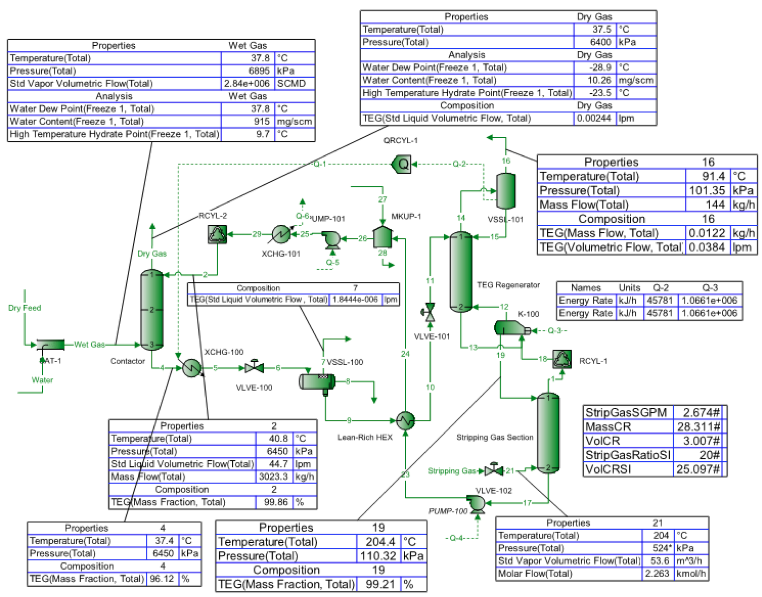Figure 1. Sample results using ProMax  for TEG dehydration with reboiler P=110.3 kPaa (16 psia) with NR=2 and NS=2

The regenerator top temperatures are exactly the same as those in Figures  2 and 3 presented in the November 2013 TOTM .

Figure 2 presents the variation of the TEG vaporization losses from still/regeneartor column top with circulation ratio (mass basis) and stripping gas rate at top pressure of 101.3 kPaa (14.7 psia) and reboiler pressure of 110.3 kPaa (16 psia) operating at 204.4°C (400°F).

As was discussed in the November  and September  2013 TOTMs, regeneration of TEG at higher reboiler pressure has several advantages such as preventing the emission of harmful contaminants like benzene, toluene, ethylbenzene, xylenes (BTEX), and hydrogen sulfide to the environment. Therefore, similar diagrams as shown in Figure 2 were generated for top pressure of 515.2 kPaa (74.7 psia) and reboiler pressure of 524.1 kPaa (76 psia) at 204.4°C (400°F). Figure 3 presents the variation of TEG vaporization loss from regenerator top for such a high reboiler pressure.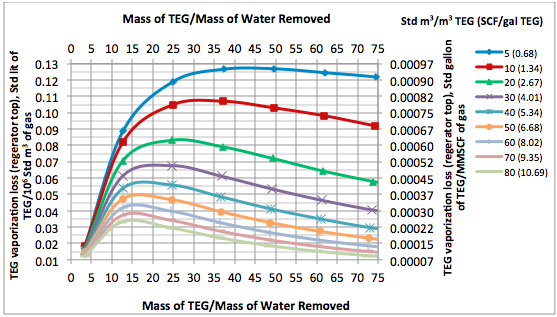Fig 2. Variation of TEG vaporization loss from regenerator top with circulation mass ratio and stripping gas rate at top P=101.3 kPaa (14.7 psia) and reboiler P=110.3 kPaa (16 psia) at 204.4°C (400°F) and NS=0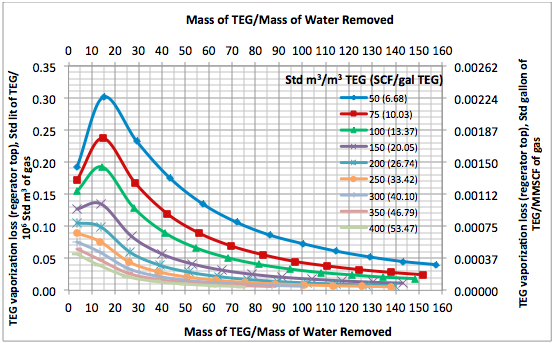Fig 3. Variation of TEG loss from regenerator top with circulation msss ratio and stripping gas rate at top P=515.2 kPaa (74.7 psia) and reboiler P=524.1 kPaa (76 psia) at 204.4°C (400°F) and NS=0

Figures 2 and 3 can be used for a quick estimate of the TEG vaporization loss from regenerator top for a given stripping gas rate and TEG circulation ratio either at low or high reboiler pressure. The two reboiler pressures selected in this study are typical operating pressures. For generation of data for Figures 2 and 3, the stripping gas was sparged directly into the reboiler; therefore,  the number of theoretical trays for stripping gas section is zero (NS=0). The corresponding figures in terms of TEG circulation volume ratio are presented in the Appendix (Figures 2A and 3A). Figures 2 and 3 indicate that as the stripping gas ratio increases the TEG vaporization losses decreases. These two figures also indicate that as the TEG mass circulation ratio increases, the TEG vaporization losses increases initially followed by a decreasing trend.

Generally, either 0, 1, or 2 ideal trays in the stripping gas section is used. In order to investigate the effect of the number of theoretical trays in the stripping gas section (NS) on the TEG vaporization loss, simulations were performed for the cases of NS=0 and NS=2 for a constant stripping gas rate. Figure 4 presents the results of these simulations for low reboiler presssures of  110.3 kPaa (16 psia). The reboiler temperature for all cases  was set at 204.4°C (400°F).

Figure 4 clearly indicates that the TEG vaporization loss from the regenerator top is independent of the number of ideal trays in the gas stripping section at low TEG mass circulation rates, however, it increases slightly with the increase in the number of ideal trays in the stripping gas section at higher TEG mass circulation ratio.

The effect of feed gas temperature to contactor and mass circulation ratio on TEG vaporization loss from the regenerator top is demonstrated in Figure 5. The TEG vaporization losses for three feed gas temperatures to contactor for stripping gas rate of 10 Std m3/m3 TEG (1.34 SCF/gal TEG) are plotted as a function of TEG mass circulation ratio. This figure indicates that as the contactor temperature increases, the TEG vaporization loss from the regenerator top increases. This can be explained because as the feed gas temperature increases, the feed gas water content (mass per unit volume at standard conditions) increases drastically which results in more vaporization of water from regenerator top. Consequently, more TEG (along with water) per unit volume of the gas at standard conditions is vaporized.

As expected Figure 6 indicates that the TEG vaporization loss from the contactor top is practically independent of the stripping gas rate. In addition this figure shows that as the TEG mass circulation ratio increases beyond 15 mass of TEG/mass of water removed, the TEG losses remain constant. As shown in Figure 7, Similar diagrams for higher reboiler pressure revealed almost the same observations.

Figure 8 also shows that as the TEG mass circulation ratio increases beyond 15 mass of TEG/mass of water removed, the TEG losses remain constant. However, as the feed gas temperature to the contactor increases, the TEG losses from the contactor top increase drastically.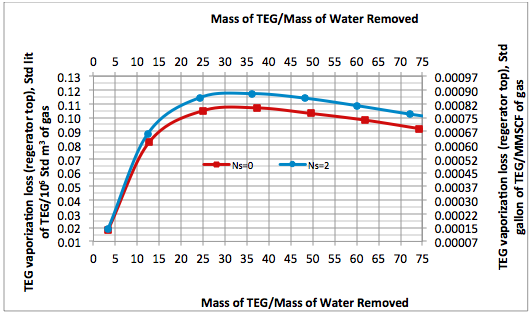Fig 4. Effect of number of ideal trays (NS) in the gas stripping section on the TEG vaporization loss from regenerator top at P=101.3 kPaa (14.7 psia), reboiler P=110.3 kPaa (16 psia) at 204.4°C (400°F) and stripping gas rate of 10 Std m3/m3 TEG (1.34 SCF/gal TEG)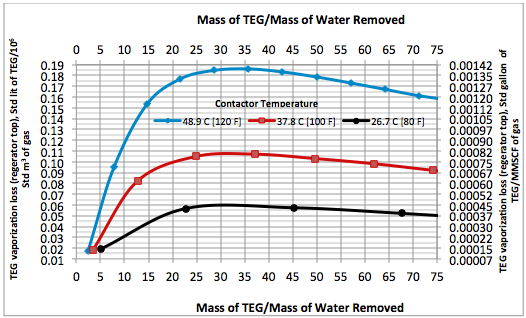Fig 5. Effect of contactor temperature and mass circulation ratio on TEG vaporization loss from regenerator top at P=101.3 kPaa (14.7 psia) and reboiler P=110.3 kPaa (16 psia) at 204.4°C (400°F), NS=0 and stripping gas rate of 10 Std m3/m3 TEG (1.34 SCF/gal TEG)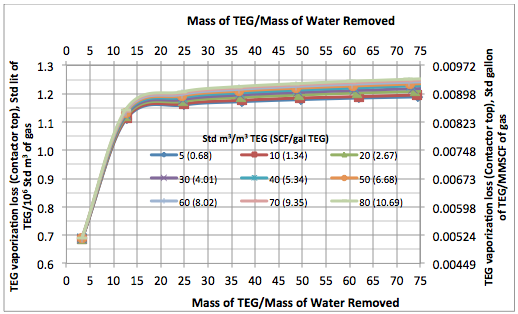Fig 6. Variation of TEG vaporization loss from contactor top with circulation mass ratio and stripping gas rate at top P=101.3 kPaa (14.7 psia) and reboiler P=110.3 kPaa (16 psia) at 204.4°C (400°F) and NS=0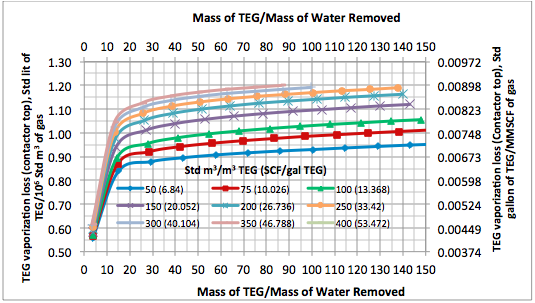Fig 7. Variation of TEG vaporization loss from contactor top with circulation mass ratio and stripping gas rate at top P=515.2 kPaa (74.7 psia) and reboiler P=524.1 kPaa (76 psia) at 204.4°C (400°F) and NS=0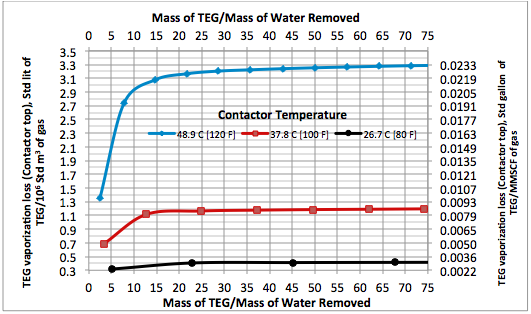Fig 8. Effect of contactor temperature and mass circulation ratio on TEG vaporization loss from contactor top at P=6897 kPaa (1000 psia), reboiler P=110 kPaa (16 psia) at 204.4°C (400°F) , NS=0 and stripping gas rate of 10 Std m3/m3 TEG (1.34 SCF/gal TEG)

Conclusions:

In this TOTM, the effect of circulation ratio, stripping gas rate, theoretical number of trays, and the feed gas temperature to the contactor column on the TEG vaporization losses from contactor  top and regenerator top for regeneration of TEG concentration at low and high reboiler pressure operating at 204.4°C (400°F) was studied. Charts for a quick estimation of the TEG vaporization losses from still/regenerator column top and contactor top at a specified stripping gas rate and circulation ratio to achieve a desired level of lean TEG concentration were prepared and presented in Figures 2, 3, 6, and 7. These charts are based on the rigorous calculations performed by computer simulations and can be used for facilities type calculations for evaluation and trouble shooting of an operating TEG dehydration unit. In addition, the following observations were made for the cases studied in this TOTM:

1. The TEG vaporization loss from the contactor top is almost 10 times higher than still/regenerator column top (see Figures 2, 3, 6 and 7).
2. As the feed gas temperature to the contactor column increases, the TEG vaporization loss from top of both columns increases (Figures 5 and 8).
3. The TEG vaporization loss from top of still/regenerator column is practically independent of the number of theoretical trays in the stripping gas section (NS), see Figure 4.
4. Pressurized reboiler results in higher TEG vaporization losses from regenerator due to higher stripping gas requirements.
5. Even though not studied in this TOTM, mechanical losses such as entrainment from contactor top and regenerator top as well as leaks from pump seals are much higher than the vaporization losses presented here.

To learn more, we suggest attending our G40 (Process/Facility Fundamentals)G4 (Gas Conditioning and Processing)G5 (Gas Conditioning and Processing-Special), and PF81 (CO2 Surface Facilities)PF4 (Oil Production and Processing Facilities)courses.

John M. Campbell Consulting (JMCC) offers consulting expertise on this subject and many others. For more information about the services JMCC provides, visit our website at www.jmcampbellconsulting.com, or email us atconsulting@jmcampbell.com.

By: Dr. Mahmood Moshfeghian

References:

1. ProMax 3.2, Bryan Research and Engineering, Inc., Bryan, Texas, 2013.
2. Soave, G., Chem. Eng. Sci. Vol. 27, No. 6, p. 1197, 1972.
3. Moshfeghian, M., http://www.jmcampbell.com/tip-of-the-month/2013/11/estimating-still-column-top-temperature-in-teg-dehydration-unit/, Tip of the Month, November 2013.
4. Moshfeghian, M., http://www.jmcampbell.com/tip-of-the-month/2013/09/high-pressure-regeneration-of-teg-with-stripping-gas/, Tip of the Month, September 2013.

Appendix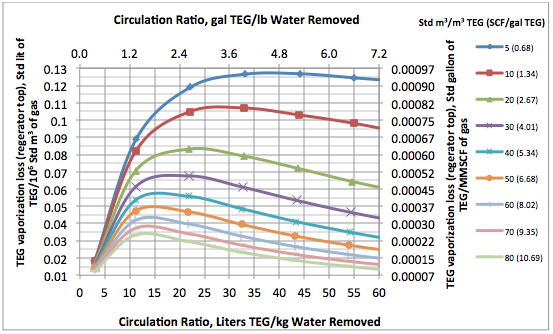Fig 2A. Variation of TEG vaporization loss from regenerator top with circulation volume ratio and stripping gas rate at top P=101.3 kPaa (14.7 psia) and reboiler P=110.3 kPaa (16 psia) at 204.4°C (400°F) and NS=0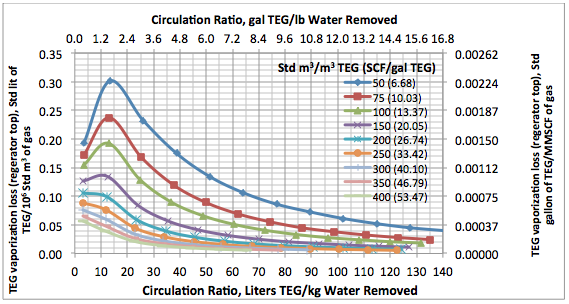Fig 3A. Variation of TEG loss from regeneartor top with circulation volume ratio and stripping gas rate at top P=515.2 kPaa (74.7 psia) and reboiler P=524.1 kPaa (76 psia) at 204.4°C (400°F) and NS=0